A question about BJT Astable Multivibrators

samy555

Joined May 24, 2010
116
HelloI read under Title "Astable Operation "I could not understand what is underlined. I understand how each transistor turns on but I could not understand how it becomes OFF

Thanks to everyone who tries to help

Jony130

Joined Feb 17, 2009
5,287

AnalogKid

Joined Aug 1, 2013
9,614
Two quickie rules of EE:
1. The voltage across a capacitor cannot change instantaneously.
2. The current through an inductor cannot change instantaneously.

When TR2 conducts, its collector goes from +9V to 0V, and change of -9V. This appears on the other side of C2 as a voltage change from whatever it was to whatever it was minus 9V. Since it was sitting at the Q1 base-emitter voltage (+0.6V), the capacitor drives the base to -8.4V. In the real world the base-emitter junction goes into reverse conduction like a zener diode at around -6V to -8V, "catching" the capacitor spike. If this circuit were run on 24V, this could be a problem because if the capacitors are big enough they could dump damaging currents through the reverse biased junctions.

ak

samy555

Joined May 24, 2010
116
Take a look here

Assuming that C1 is not initially charged and Q1 is ON so Q2 must be OFF since voltage on C1 is 0V (empty capacitor act like short-circuit) and Q1 is in saturation (Vce≈0V)So voltage on base Q2 is near 0V and that why Q2 is cut-off.
C2 is quickly charge to 8.4V through:
Vcc-->R4--->C2--->base-emiter Q1-->gnd (blue line)
But Q1 is still on saturation thanks to R3 (purple line)

And C1 begins to charge through
Vcc-->R2-->C1-->collector-emitter Q1--->gnd (red line)
When voltages on C1 reach 0.6V Q2 is now begins to open.
Opening Q2 means that voltage on collector Q2 start to drop.
OK,,, now we have C1 voltage around 0.6
C2 voltage around 8.4
Q1 was ON
Q2 starts to be ON
Since capacitor C2 has been previously charged to the voltage around 8.4V. This voltage on C2 cannot suddenly change (charged capacitor act as a voltage source),
OK I get it
so when the collector voltage of Q2 and "positive" electrode of C1 drops to zero, the "negative" plate of C1 decrease below the GND to -8.4V. So voltage on Q1 base becomes negative -8.4V, and that cut-off Q1.
You say that when VCE2 = 0 and the right side plate of C1 = 0 (I don't know how right side plate of C1 = 0 !!??)
I can not understand

samy555

Joined May 24, 2010
116
Two quickie rules of EE:
1. The voltage across a capacitor cannot change instantaneously.
2. The current through an inductor cannot change instantaneously.
OK I know that

When TR2 conducts, its collector goes from +9V to 0V,
Yes of course you are right
and change of -9V.
Yes, Change = 0-9 = -9 (mathimatically true but I cann't imagine it)
This appears on the other side of C2 as a voltage change from whatever it was to whatever it was minus 9V.
Since it was sitting at the Q1 base-emitter voltage (+0.6V), the capacitor drives the base to -8.4V. In the real world the base-emitter junction goes into reverse conduction like a zener diode at around -6V to -8V, "catching" the capacitor spike. If this circuit were run on 24V, this could be a problem because if the capacitors are big enough they could dump damaging currents through the reverse biased junctions.

ak
I cann't understand

Jony130

Joined Feb 17, 2009
5,287
You say that when VCE2 = 0 and the right side plate of C1 = 0 (I don't know how right side plate of C1 = 0 !!??)
I can not understand
Charged capacitor act just like a voltage source. So if we charge a capacitor to say 8.4V and now we "short" a right plate via transistor to GND.
What will be the voltage at the negative plate ??
The situation is exactly the same as in this circuit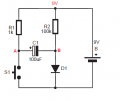Where S1 represent the transistor collector-emitter "junction".

At the beginning the capacitor is empty so Vab = 0V. But at the moment when we connect a power supply the current will start to flow and the situation look like this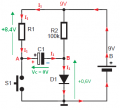The charging current is flowing and the voltage across is rising with the time constant R1*C1.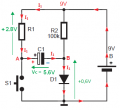And after t = 5R1*C1 the capacitor is fully charged which means that I1 = 0A but Vab = 8.4V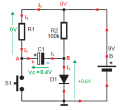And now we push the switch. This means that we short the left plate of a capacitor directly to GND. But our capacitor was previously charged to the voltage around 8.4V. So the capacitor will act just like a battery. And we connect the positive terminal of this battery to GND.
So the voltage at the negative terminal (node B) with respect to ground is now equal to -8.4V.
This pic show the details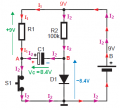and as capacitor discharge the voltage across capacitor drop.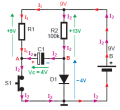•samy555, absf and atferrari

crutschow

Joined Mar 14, 2008
28,474
In a nutshell, when the voltage on one end of a capacitor changes instantly (or nearly so) by X volts, the voltage on the other end changes by X volts also.
This is independent of the original voltage at each end of the cap.
Follow that rule and you'll understand the circuit.

•absf

samy555

Joined May 24, 2010
116
And now we push the switch. This means that we short the left plate of a capacitor directly to GND. But our capacitor was previously charged to the voltage around 8.4V. So the capacitor will act just like a battery. And we connect the positive terminal of this battery to GND.
So the voltage at the negative terminal (node B) with respect to ground is now equal to -8.4V.
This pic show the details

View attachment 91697
Thank you very much, and in all languages of the world
I understood every letter you said but unfortunately I did not understand the above part, which is the same part that I could not understand all the time.

But wait a minute I see it another way, the voltage between point A and GND when the switch S1 is pushed = zero, so if 8.4V was on the capacitor this means that -8.4V must be on the diode (Total = 0)
Thank you jony130

Your answers always be distinct and I benefited a lot of them

samy555

Joined May 24, 2010
116
In a nutshell, when the voltage on one end of a capacitor changes instantly (or nearly so) by X volts, the voltage on the other end changes by X volts also.
This is independent of the original voltage at each end of the cap.
Follow that rule and you'll understand the circuit.
Thank you
please give me a numerical example

crutschow

Joined Mar 14, 2008
28,474
Thank you
please give me a numerical example
OK.
One end of a cap is at 0V and the other end is 9V (capacitor charged to 9V).
If the 9V end is suddenly grounded, the other end of the cap will instantly go to -9V.

Last edited:
•samy555

Jony130

Joined Feb 17, 2009
5,287
Thank you very much, and in all languages of the world
But this has nothing to do with capacitors.
What is the voltage at point B with respect to GND for this circuit: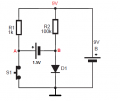But I'm glad that you finally got it.

Thank you
please give me a numerical example
Let's take this case as a exampleVoltage at point A is equal to VA = 6.2V and VB = 0.6V . And now if we press the S1 switch the VA voltage will changes instantly from 6.2V into 0V (0V - 6.2V = -6.2V), the voltage on the other end of a capacitor will also changes instantly by 6.2 volts. In this case from +0.6V into -5.6V (0.6V - 6.2V = -5.6V )

•samy555